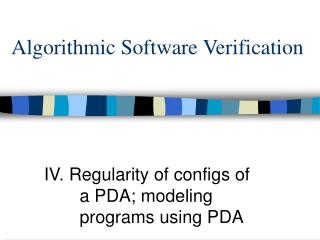# Algorithmic Software Verification - PowerPoint PPT PresentationDownload PresentationAlgorithmic Software Verification

Algorithmic Software Verification
Download Presentation## Algorithmic Software Verification

- - - - - - - - - - - - - - - - - - - - - - - - - - - E N D - - - - - - - - - - - - - - - - - - - - - - - - - - -
##### Presentation Transcript

1. Algorithmic Software Verification IV. Regularity of configs of a PDA; modeling programs using PDA

2. Pushdown automata (, Q, q_in, E, F) Q – finite set of states q_in -- initial state  -- stack alphabet E: { q –a, push d  q’ a , e   q –a, pop d  q’ q –a  q’ }

3. Configurations A = (, Q, q_in, E, F) Configuration of A: (q, w) -- q  Q, w  * Configuration graph of A: (q, w)  (q’, w) if there is a trans q –a q’ (q, w)  (q’, w d) if there is a trans q –a,push dq’ (q, w d)  (q’, w) if there is a trans q –a,pop dq’ Note that this is an infinite graph. A config (q,w) is reachable if there is a path from (q_in, ε) in the config graph.

4. Configurations A = (, Q, q_in, E, F) Let CA = { wq | (q,w) is a reachable config } We want to show that CA is regular. Also, the size of the automaton accepting CA is cubic in |A|.

5. Automaton accepting CA A = (, Q, q_in, E, F) We will construct automaton B accepting CA Let states of CA be Q. Alphabet of CA is ; initial state is q_in; final states: Q Edges: The smallest set of edges such that: • If q –a q’ then q – ε q’ must belong to B • If q –a,push dq’ then q –d q’ must belong to B • If q –a, pop d  q’ and q’’ --a* q then q’’ – ε q’ must belong to B

6. Constructing B in O(n^3) time A = (, Q, q_in, E, F) stack:= emptyset; hash:= emptyset; for every q in Q, put (q,q) on stack for every q,q’, set (q, q’).c-direct := emptyset; (q, q’).c-trans := emptyset; for every transitions u –push a q, q –pop a v put (u,v) on (q,q’).c-direct for every q, q’ in Q, q diff from q’, for every t in Q, put [ (q’, t)  (q, t) ] and [ (t, q)  (t, q’) ] in (q,q’).c-trans while stack is not empty e:= pop(stack) put e in hash (if e is not already in hash) transfer e.c-direct into stack for every [ f  g ] in e.c-trans if f is in hash, then put g on stack else put g on f.c-direct

7. Constructing B in O(n^3) time Stack – unprocessed epsilon edges Hash -- processed epsilon edges (q,q’).c-direct has (u,v): if (q, q’) is an eps edge, then (u,v) is an eps edge too. (q,q’).c-trans has (u1, v1)  (u2, v2): Given eps transition (q,q’), if (u1, v1) is an epsilon edge, then (u2, v2) is also an epsilon edge.

8. Emptiness L(A) is nonempty if and only if there is some reachable configuration of the form (q_f, w), q_f in F if and only if q_f is reachable from q_in Hence L(A) emptiness can be decided in O(n^3) time

9. Homework II 1. Take the translation of PDA emptiness to finite state games. This works only if emptiness of PDA is with respect to empty stack: i.e. PDA must read a word and at the end have an empty stack in order to accept the word. Modify the translation such that it works for PDA that accept by finite state. [ You are not allowed to transform the PDA into one accepting by empty stack ]

10. Homework II 2. Take any reasonable non-empty PDA. Translate the PDA emptiness problem into a game, solve the game and find the winning strategy. Then, give the word(s) that are accepted by the PDA which correspond to the winning strategy you have chosen. (PDA should be reasonable – should not be regular!)